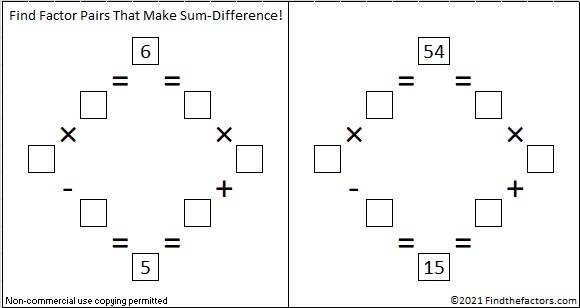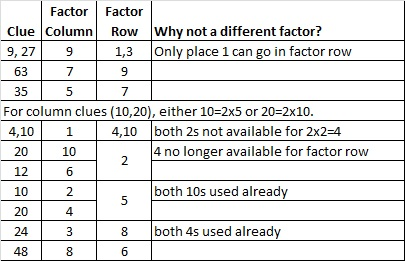# 54 and How Many Squares Are in This Puzzle?

Contents

### How Many Squares?

How many squares are in this puzzle? Finding that answer is too tedious for me to pursue. There is 1 square that is bigger than all the rest, 169 of the smallest size squares, and some different number between 1 and 169 for each size square in between. Also, I think the clues seem to form three little squares, and one of the clues, 16, happens to be a perfect square. It’s a trick question, and most people don’t like trick questions!

Solving the actual puzzle will actually be less work and more fun. The actual puzzle challenges you to write the numbers 1 through 12 in the top row and again in the first column so that the answers you write and the clues inside the puzzle work together as a multiplication table. Use logic to find the unique solution to the puzzle.This week’s puzzles are available in an excel file here. If you have a spreadsheet program on your computer, you can access it. If you enable editing in excel, you can type your answers directly onto the puzzle, and you can also easily print the puzzles.

### Factors of 54:

54 is a composite number. 54 = 1 x 54, 2 x 27, 3 x 18, or 6 x 9. Factors of 54: 1, 2, 3, 6, 9, 18, 27, 54. Prime factorization: 54 = 2 x 3 x 3 x 3, which can also be written 2 x 3³.Sometimes 54 is a clue in the FIND THE FACTORS puzzles. Even though it has other factors, the only multiplication fact the puzzle uses is 6 x 9 = 54.

### Sum-Difference Puzzle:

The number 6 has two factor pairs. One of those factor pairs adds up to 5, and the other one subtracts to 5. Can you put those factors in the correct places in the first puzzle?

The number 54 has four factor pairs. One of those factor pairs adds up to 15, and another one subtracts to 15. If you can identify those factor pairs, then you can solve the second puzzle.The second puzzle is really just the first puzzle in disguise. Why would I say that?

###Here is one way those factors can be found using logic.## 2 thoughts on “54 and How Many Squares Are in This Puzzle?”

1.fluffy

If I understand the opening question correctly (the number of NxN squares that you can derive from the grid squares of the puzzle itself), it’s pretty simple; there’s 1 13×13, 4 12×12, 9 11×11, 16 10×10, etc., all the way up to 169 1×1. 819 in all.

2.ivasallay

You make it sound so easy! I wasn’t aware there was an algorithm to find the answer, but I shouldn’t be surprised there is one. Thanks for enlightening me.

This site uses Akismet to reduce spam. Learn how your comment data is processed.